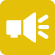## 1-Minute Survey

What Type of Investor Are You? – Take This 1-Min Survey to Find Out

Financial Literacy

# The Black Scholes Model: An Options Pricing Formula

The Black Scholes Model is a mathematical options-pricing model used to determine the prices of call and put options. The standard formula is only for European options, but it can be adjusted to value American options as well.

This mathematical formula is also known as the Black-Scholes-Merton (BSM) Model, and it won the prestigious Nobel Prize in economics for its groundbreaking work in pricing options.

In this article, we will look at the basics of the formula to gain a better understanding of what it is and how it works. By the end of this article, you should have a foundation in how to price options using the Black Scholes Model.## An Introduction to the Black Scholes Model

The Black-Scholes-Merton Model was created by three economists, Fischer Black, Myron Scholes and Robert Merton (hence its name), and it became the first widely used options pricing equation and arguably the most famous.

The mathematical model first appeared in the 1973 paper “The Pricing of Options and Corporate Liabilities” in The Journal of Political Economy, authored by Black and Scholes.

In an unfortunate bit of timing, Black died two years before Scholes and Merton received the 1997 Nobel Prize, and the award is not given posthumously. However, the committee acknowledged the essential role that Fischer Black played in the creation of the Black Scholes Model.

The formula prices options using several different factors, one of the most important of which is the constant price variation of the underlying security, or its price volatility.

Other factors important to the model include the following:

• Strike price
• Time value of money
• Expected interest rates
• Expected volatility
• Current stock prices
• Expected dividends
• Time to expiration.

## Assumptions of the Black Scholes Model

The Black Scholes Model rests on a number of assumptions to properly price options contracts:

### 1. Transaction Costs

The equation assumes that there are no transaction costs associated with purchasing the contract. Generally, this assumption is more likely to be true today than when the model was developed, thanks in large part to platforms like Robinhood and others that don’t charge high fees or commissions for options trades.

### 2. European Options

The formula assumes that options are European-style only. European options are those that can be exercised only on their expiration dates, while American options may be exercised anytime during the life of the contract, through the expiration date.

### 3. Interest Rates and Volatility

The Black Scholes Model takes into account current interest rates and expected volatility. In order to do so, it assumes that these elements – including the risk-free interest rate – are known and constant throughout the life of the contract.

### 4. The Efficient Market Theory

The model assumes that the Efficient Market Theory – a hypothesis that all available information is currently priced into the market, thus making market movements random and unpredictable – is true.

### 5. Dividends

The formula assumes that the underlying security doesn’t pay dividends during the life of the option. However, some analysts adapt the formula to take dividends into consideration. In general, it can be adapted if you know the price of the underlying stock on the ex-dividend date.

## The Black Scholes Model’s Formula

The formula may look intimidating, but here’s the good news: You don’t really need to understand the underlying math to use it for options pricing.

Generally, there are tools you can use that can help you calculate prices. These include spreadsheets, trading platforms and online calculators. You can find them with a simple Google search. For a more generalized tool though, check out this free investment calculator.

You can write the Black Scholes formula in different ways, including this one: C = S(t​) N(d1) Ke^(rt) x N(d2)

Intimidating, yes. But here’s how it all shakes out:

C = Price of the call option
S = Current stock (underlying asset) price
K = Strike rice
r = Risk-free interest rate
t = Time to maturity
N = Normal distribution

D1 and d2 are variables that you calculate based on underlying equations. D1 is a logarithmic equation that takes into account expected volatility and d2 is an equation derived from d1 and the time to maturity.

Again, while the math is complicated, you don’t need to fully understand it to make use of the formula in your options strategies. You can use the variety of tools out there to perform the calculations for you.

## Concluding Thoughts on the Black Scholes Model

The Black Scholes Model is definitely complicated. But it’s certainly a useful and respected way to calculate the prices of options. Specifically, it’s used most essentially to calculate the prices of European-style call options using some default assumptions.

If you enjoyed this article on the Black Scholes Model, check out the next article in our series on options trading, “Selling Put Options.”

Brian M. Reiser has a Bachelor of Science degree in Management with a concentration in finance from the School of Management at Binghamton University.

He also holds a B.A. in philosophy from Columbia University and an M.A. in philosophy from the University of South Florida.

His primary interests at Investment U include personal finance, debt, tech stocks and more.

###### Articles by Brian M. ReiserInvestment Opportunities
###### Can Mobile Esports Company Skillz Inc.’s IPO Level Up for Investors?

December 18, 2020